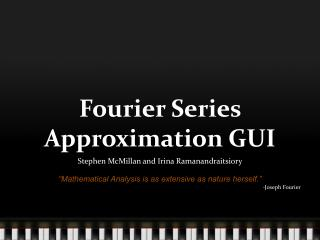DownloadDownload PresentationFourier Series Approximation GUI

# Fourier Series Approximation GUI

Télécharger la présentation## Fourier Series Approximation GUI

- - - - - - - - - - - - - - - - - - - - - - - - - - - E N D - - - - - - - - - - - - - - - - - - - - - - - - - - -
##### Presentation Transcript

1. Fourier Series Approximation GUI Stephen McMillan and Irina Ramanandraitsiory “Mathematical Analysis is as extensive as nature herself.” -Joseph Fourier

2. Joseph Fourier • French mathematician (21 March 1768 – 16 May 1830) • One of his most noteworthy achievements was the formulation of the Fourier Series. • Alongside his mathematical contributions he is credited as the first to propose the concept of the greenhouse effect.

3. The Fourier Series • A mathematical tool used to decompose any arbitrary periodic signal or function into a possibly infinite set of simple sine and cosine functions. • Originally discovered to help Fourier with the heat equation in a metal plate. • Developed off of former work done by Leonhard Euler, Daniel Bernoulli, and others on trigonometric series.

4. Mathematical Definition - James Stewart

5. Our Goal • Make an educational labview GUI that allows the user to experiment with the Fourier Series in order to gain an understanding of its power and usefulness.

6. GUI Features • Allows the user to try and approximate a square, triangle, or saw tooth wave (of any amplitude and frequency) by modifying the amplitude and frequency of 5 sine waves. • Plots each modification in real time. • Shows the time response and the frequency response. • Shows the effects of a low pass filter and a band pass filter. • Allows for automatic approximations in case the user has trouble finding the correct amplitudes and frequencies.

7. Example Front Panel

8. Example (cont.) Time Response of the Summed Sine Waves

9. Example (cont.) Frequency Response of the Summed Sine Waves

10. Example (cont.) Effect of the Low Pass Filter on the Summed Sine Waves

11. Example (cont.) Effect of the Band Pass Filter on the Summed Sine Waves

12. Applications of the Fourier Series • Led to the development of the Fourier Transform which decomposes non-periodic function. • Used in file compression such as JPEG image format. • Used in signal processing in communications and astronomy, acoustics, optics, and cryptography.

13. Sources • http://ocw.mit.edu/courses/mathematics/18-100c-analysis-i-spring-2006/projects/niu.pdf • http://en.wikipedia.org/wiki/Fourier_series • http://www.stewartcalculus.com/data/CALCULUS%20Early%20Transcendentals/upfiles/FourierSeries5ET.pdf • https://kiwi.ecn.purdue.edu/rhea/index.php/ECE_301_Fall_2007_mboutin_Definitions • http://strangewondrous.net/browse/author/f/fourier+joseph